Question

2) Let B = {(1, 3, 4), (2,-5,2), (-4,2-6)) and B/-(( 1, 2,-2), (4, 1,-4), (-2, 5, 8)) be 5 ordered bases of R2. Let x = | 8 | in the standard basis of R2. a) Use a matrix and x to find L18 ]B. b) U...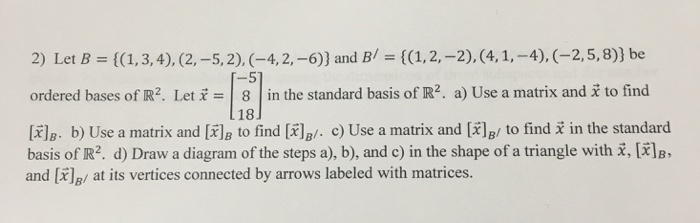2) Let B = {(1, 3, 4), (2,-5,2), (-4,2-6)) and B/-(( 1, 2,-2), (4, 1,-4), (-2, 5, 8)) be 5 ordered bases of R2. Let x = | 8 | in the standard basis of R2. a) Use a matrix and x to find L18 ]B. b) Use a matrix and [X]B to find [x)B/. c) Use a matrix and [X]B/ to find x in the standard basis of R2, d) Draw a diagram of the steps a), b), and c) in the shape of a triangle with x, [ ]B, and [lg/ at its vertices connected by arrows labeled with matrices.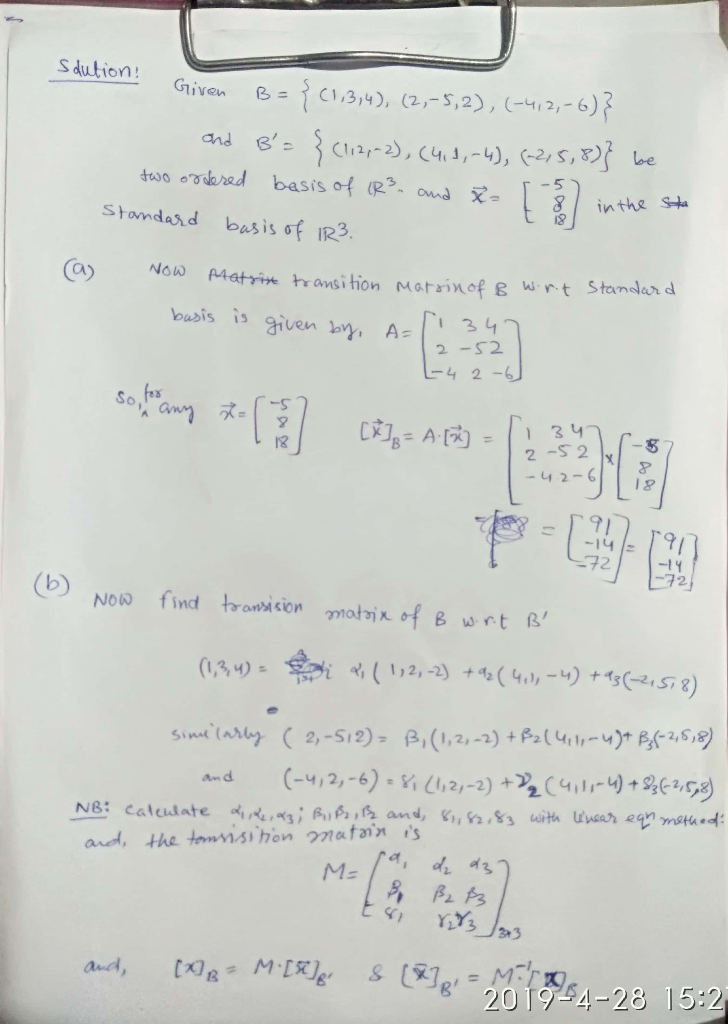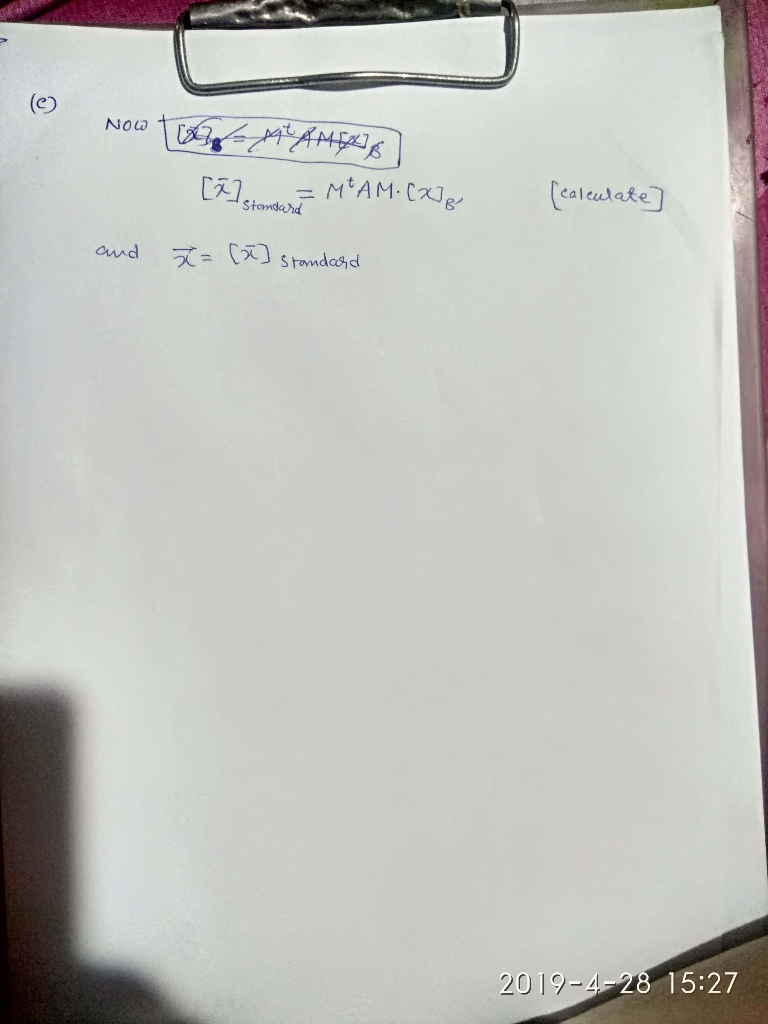Earn Coins

Coins can be redeemed for fabulous gifts.

Similar Homework Help Questions
• 2 question ---------------------------------------------------------- (1 point) Consider the ordered bases B =( (8-4] [: • and c-...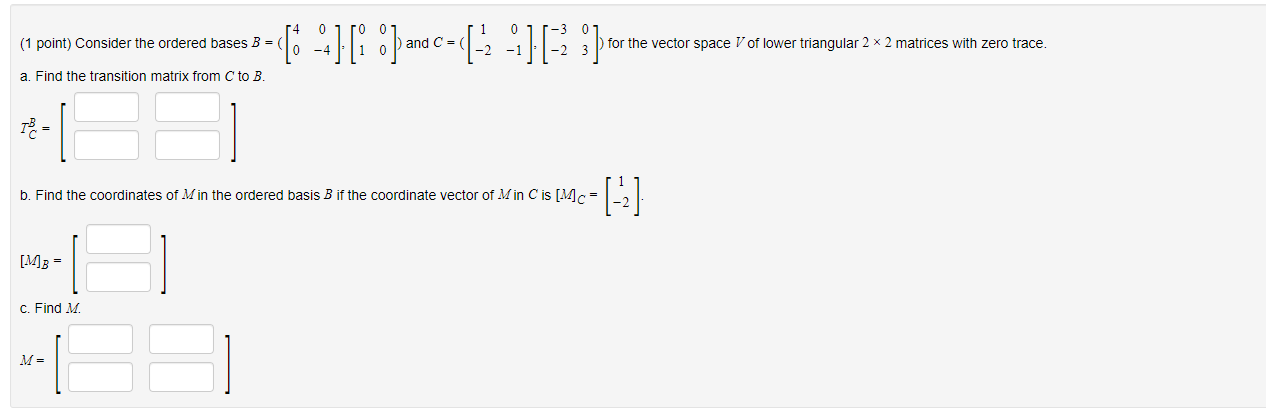2 question ---------------------------------------------------------- (1 point) Consider the ordered bases B =( (8-4] [: • and c- (- -)( :} ) for the vector space V of lower triangular 2 x 2 matrices with zero trace. a. Find the transition matrix from C to B. TB = b. Find the coordinates of Min the ordered basis B if the coordinate vector of Min C is [Mc= [MB = C. Find M. M= (1 point) Consider the ordered bases B [ 1...

• Find the matrix A' for T relative to the basis B'. T: R2 + R2, T(x,...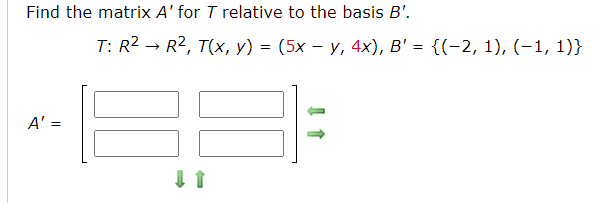Find the matrix A' for T relative to the basis B'. T: R2 + R2, T(x, y) = (3x - y, 4x), B' = {(-2, 1), (-1, 1)} A' = Let B = {(1, 3), (-2,-2)} and B' = {(-12, 0), (-4,4)} be bases for R2, and let 0 2 A = 3 4 be the matrix for T: R2 + R2 relative to B. (a) Find the transition matrix P from B' to B. 6 4 P= 9 4...

• {(1,3), (2,-2)} and B = {(-12,0), (-4, 4)} be the basis for R2 and let A...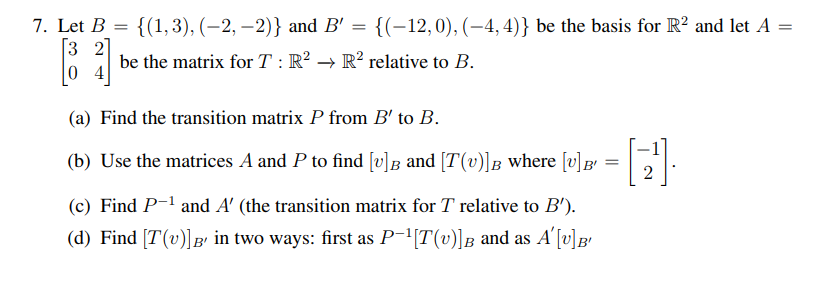{(1,3), (2,-2)} and B = {(-12,0), (-4, 4)} be the basis for R2 and let A = 7. Let B 3 2 0 4 be the matrix for T R2 -> R2 relative to B (a) Find the transition matrix P from B' to B (b) Use the matrices A and P to find [v]B and [T(v)]B where v] 2 (c) Find P and A' (the transition matrix for T relative to B') (d) Find [T(v)B' in two ways: first...

• (1 point) Consider the ordered bases B = a. Find the transition matrix from C to...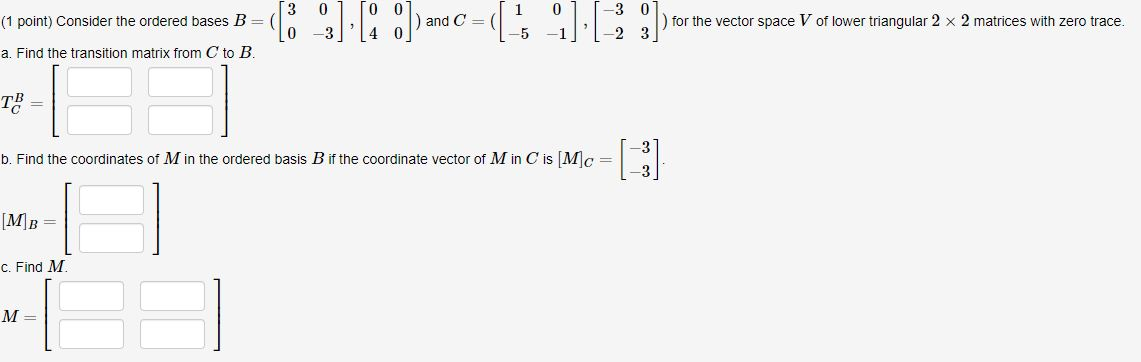(1 point) Consider the ordered bases B = a. Find the transition matrix from C to B. 3 01 To Olmedi 011-3 0. *1 for the vector space V of lower triangular 2 x 2 matrices with zero trace. 3 4 01) and C=-5 -1/'1-23] b. Find the coordinates of M in the ordered basis B if the coordinate vector of M in C is M c [ MB = C. Find M. M =

• (1 point) Consider the ordered bases B = (1 – X,4 – 3x) and C =...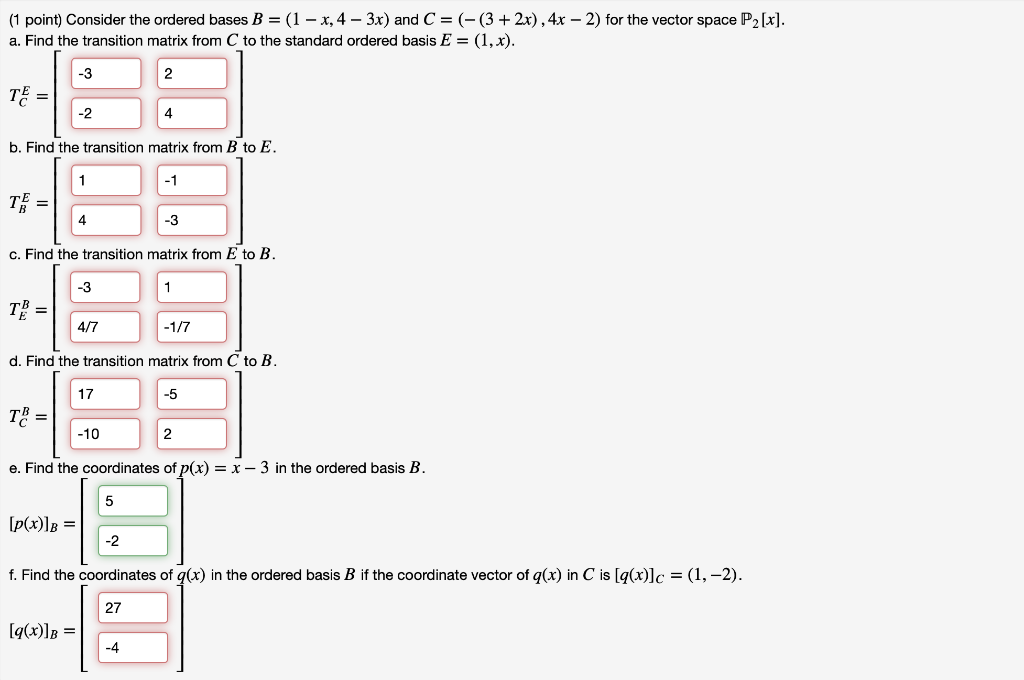(1 point) Consider the ordered bases B = (1 – X,4 – 3x) and C = (-(3 + 2x), 4x – 2) for the vector space P2[x]. a. Find the transition matrix from C to the standard ordered basis E = (1, x). -3 2 TE = -2 b. Find the transition matrix from B to E. 1 -1 T = 4 -3 c. Find the transition matrix from E to B. -3 1 T = 4/7 -1/7 d. Find...

• let T : R2[x] → R2[x] have matrix shown in the picture with respect to basis B = {1 + x, x + 2x2, 2 + x − x2}. 8. Comput...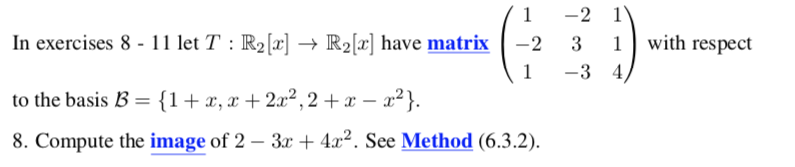let T : R2[x] → R2[x] have matrix shown in the picture with respect to basis B = {1 + x, x + 2x2, 2 + x − x2}. 8. Compute the image of 2 − 3x + 4x2. 1 -2 1 In exercises 8-1 1 let T : R2 → Rİx] have matrix |ー2 1 | with respect 3 1 -3 4 to the basis B = {1 + x, x + 22.2, 2 + x-rj 8. Compute the...

• Exercise 1. Let S(2) = (€1,6) be the standard basis of T R2 and let B...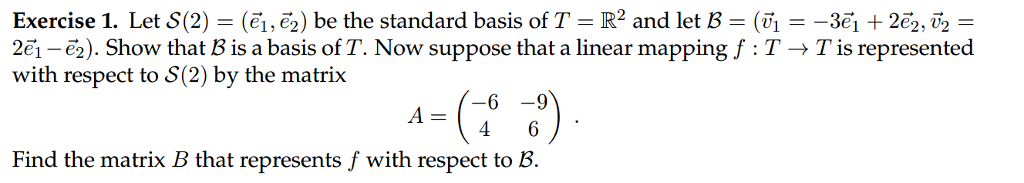Exercise 1. Let S(2) = (€1,6) be the standard basis of T R2 and let B = (? =-3e1 + 2e, v2 = 2e1-6). Show that B is a basis of T. Now suppose that a linear mapping f : T T is represented with respect to 8(2) by the matrix oSe 4 6' Find the matrix B that represents f with respect to B.

• Let 9 - {(1,3), (-2,-2)) and 8 = {(-12, 0),(-4,4) be bases for R, and let...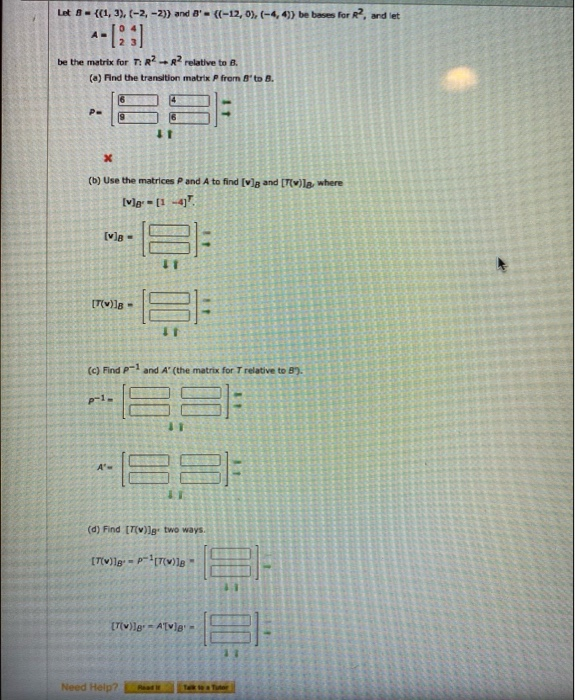Let 9 - {(1,3), (-2,-2)) and 8 = {(-12, 0),(-4,4) be bases for R, and let --12:] be the matrix for T. R2 + R2 relative to B. (2) Find the transition matrix P from 8' to B. P. X (b) Use the matrices P and A to find [v]g and [T()le, where Ivo - [1 -4 [va - [T]8 - I (c) Find p-1 and A' (the matrix for T relative to B). p-1- II A- (d) Find (TV)]g...

• Problem 4 Let T:R2 R2 be defined by and a be the standard basis for R2....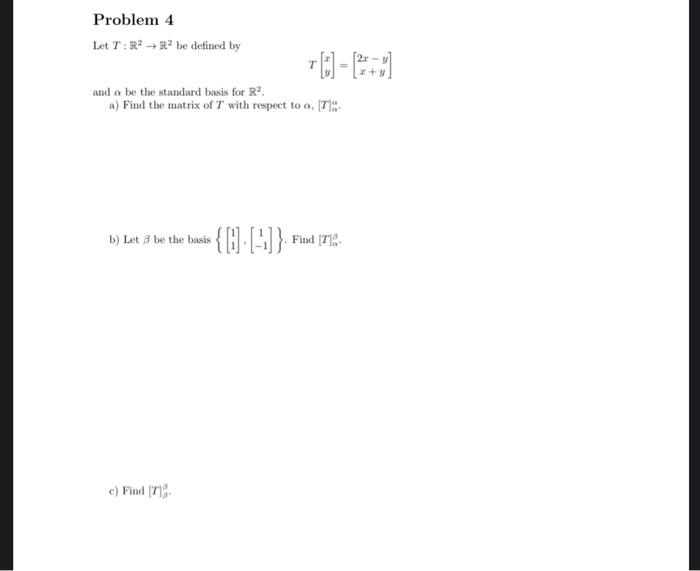Problem 4 Let T:R2 R2 be defined by and a be the standard basis for R2. a) Find the matrix of T with respect to a, (T): b) Let 3 be the basis { 1 -1}. Find (T18 c) Find (7)

• (1 point) Consider the ordered bases B = {-(7 + 3x), –(2+ x)} and C =...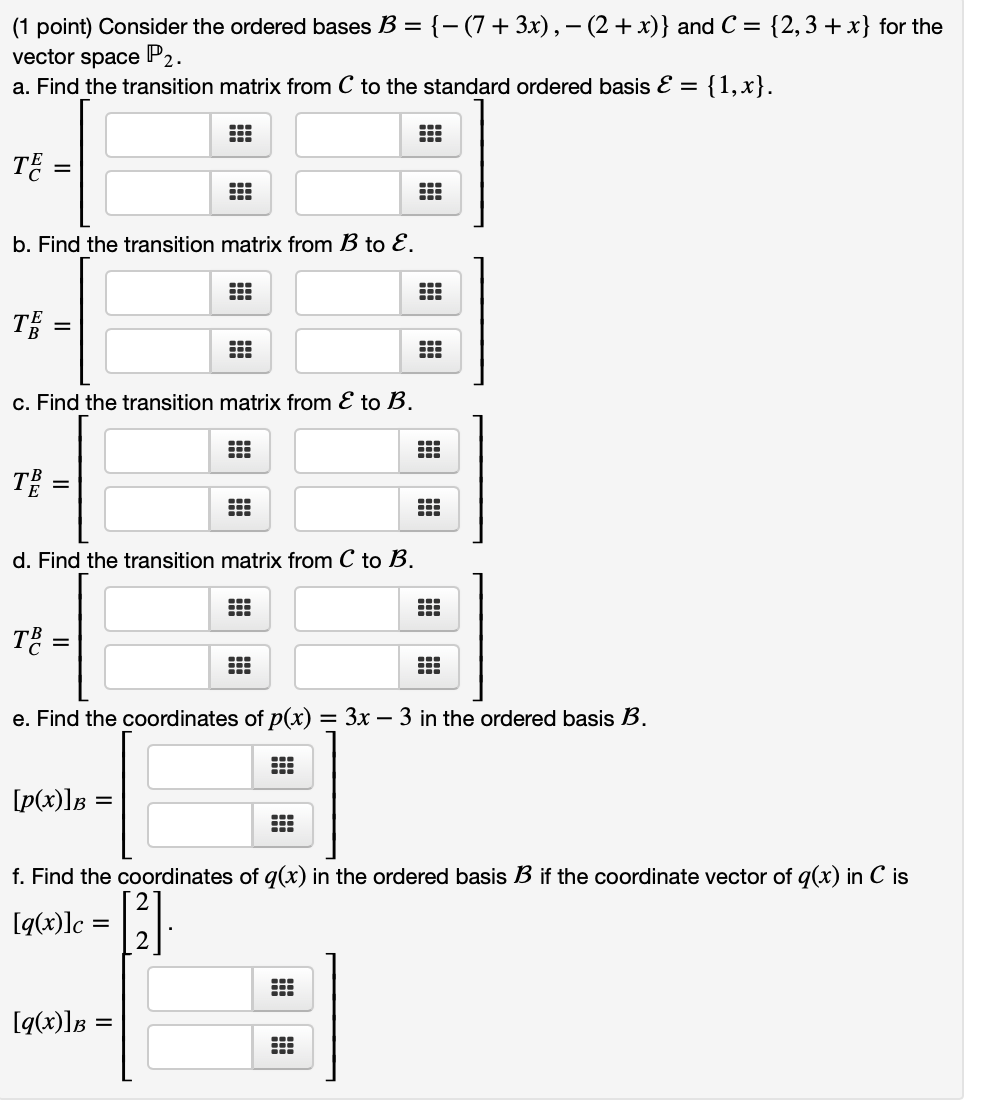(1 point) Consider the ordered bases B = {-(7 + 3x), –(2+ x)} and C = {2,3 + x} for the vector space P2. a. Find the transition matrix from C to the standard ordered basis E = {1,x}. TE = b. Find the transition matrix from B to E. Te = c. Find the transition matrix from E to B. 100 TB = d. Find the transition matrix from C to B. TB = 11. !!! e. Find the...